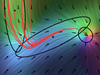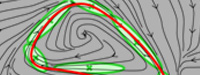### Abstract

We present a probabilistic approach to learn robust models of human motion through imitation. The association of Hidden Markov Model (HMM), Gaussian Mixture Regression (GMR) and dynamical systems allows us to extract redundancies across multiple demonstrations and build time-independent models to reproduce the dynamics of the demonstrated movements. The approach is first systematically evaluated and compared with other approaches by using generated trajectories sharing similarities with human gestures. Three applications on different types of robots are then presented. An experiment with the iCub humanoid robot acquiring a bimanual dancing motion is first presented to show that the system can also handle cyclic motion. An experiment with a 7 DOFs WAM robotic arm learning the motion of hitting a ball with a table tennis racket is presented to highlight the possibility to encode several variations of a movement in a single model. Finally, an experiment with a HOAP-3 humanoid robot learning to manipulate a spoon to feed the Robota humanoid robot is presented to demonstrate the capability of the system to handle several constraints simultaneously.

### Bibtex reference

@inproceedings{Calinon10COGSYS,
author="Calinon, S. and Sauser, E.L. and Billard, A.G. and Caldwell, D.G.",
title="A learning by imitation model handling multiple constraints and motion alternatives",
booktitle="Intl Conf. on Cognitive Systems (CogSys)",
year="2010",
month="January",
}

### Video

A HOAP-3 humanoid robot is used in the experiment. It has in total 28 DOFs, of which the 8 DOFs of the upper torso are used in the experiment. The experiment consists of feeding a Robota robotic doll, where HOAP-3 first brings a spoon to a plate of mashed potatoes and then moves it towards Robota's mouth.

This experiment aims at demonstrating that the framework can be used to learn a controller by taking into account several constraints. Here, we consider the case where a set of movements relative to a set of landmarks must be taken into consideration for the reproduction of the skill (i.e., where several actions on objects are considered).

### Source codesDownload GMR Dynamics sourcecode

#### Usage

Unzip the file and run 'demo1' in Matlab.

#### Reference

• Calinon, S., D'halluin, F., Sauser, E.L., Caldwell, D.G. and Billard, A.G. (2009) A probabilistic approach based on dynamical systems to learn and reproduce gestures by imitation. IEEE Robotics and Automation Magazine.

#### Demo 1 - Demonstration of a trajectory learning system robust to perturbation based on Gaussian Mixture Regression (GMR)This program first encodes a trajectory represented through time 't', position 'x' and velocity 'dx' in a joint distribution P(t,x,dx) through Gaussian Mixture Model (GMM) by using Expectation-Maximization (EM) algorithm. Gaussian Mixture Regression (GMR) is then used to estimate P(x,dx|t), which retrieves another GMM refining the joint distribution model of position and velocity.
The learned skill can then be reproduced by combining an estimation of P(dx|x) with an attractor to the demonstrated trajectories.